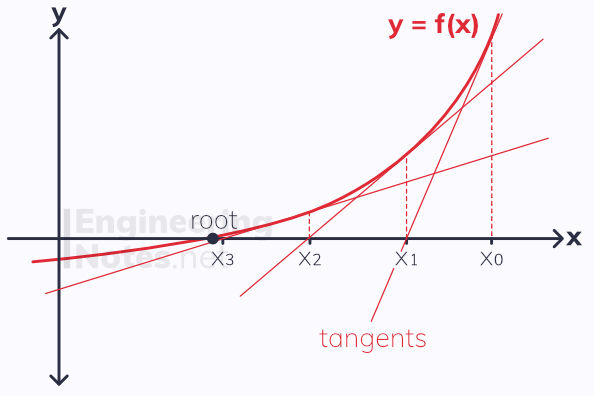top of page

#### Notes by Category University Engineering

Rate these notesNot a fanNot so goodGoodVery goodBrillRate these notes

# Numerical Methods

A root of a function is when the function = 0. On a graph, this is represented by an x-axis intersection, where y-value changes from positive to negative or vice versa. Therefore, we can locate roots by showing that the sign has changed.However, this only applies if the function is continuous throughout the given interval, notated using square brackets [a, b]. This means that the graph does not jump from one value to another, but changes smoothly. If there is a vertical asymptote in the interval, it is not continuous.

If f(x) is continuous on the interval [a, b], and f(a) and f(b) have opposite signs, then there is a root between the two

## Iteration

It is possible to estimate roots using an iterative method. Generally, the equation needs to be manipulated slightly before it works, as the linear variable (x) has to be the subject.

To solve an equation in the form f(x) = 0 using iteration, rearrange it into the form x = g(x) and apply iteration

Applying iteration means you use put the value you get out of g(x) back into the function, and repeat this multiple times.

There are multiple rearrangements for each function f(x), giving different variations of x = g(x). These different iterations will either converge to a root, or diverge:• If the iteration converges closer and closer to the root, always in the same direction, it forms a staircase diagram.

• If the iteration converges in an alternating pattern above and below the root, a cobweb diagram is formed.

• If the iteration diverges, it moves away from the root very quickly.

## The Newton-Raphson Method

The Newton-Raphson method is the method used by many calculators to solve equations in the form f(x) = 0. The formula for the Newton-Raphson method is:It works using tangent lines with increasing accuracy to approximate a root:As you can see, it relies on differentiation, and so cannot work when f'(x) equals or is close to equalling zero.• If the starting value x₀ is close to a turning point, the method does not work, because the gradient of the tangent is very small, so the tangent crosses the x-axis a long way away from x₀

• If any value of x is on a turning point, the method does not work because the gradient of the tangent is 0, and so never crosses the x-axis.

See All